# Lathyrus vernus function-based age-by-stage MPMs, LTRE and sLTRE analysis

In this vignette, we use the lathyrus dataset to illustrate the estimation of age-by-stage function-based MPMs. To reduce output size, we have prevented some statements from running if they produce long stretches of output. Examples include most summary() calls. Remove the hashtags to run these calls. This vignette is only a sample analysis. Detailed information and instructions on using lefko3 are available through a free online e-book called lefko3: a gentle introduction, available on the projects page of the Shefferson lab website.

### ORGANISM AND POPULATION

Lathyrus vernus (family Fabaceae) is a long-lived forest herb, native to Europe and large parts of northern Asia. Please see our description of the plant, study site, and methods in our vignette on raw ahistorical MPM creation and analysis.

### BASIC WORKFLOW

The dataset that we have provided is organized in horizontal format, meaning that each row holds all of the data for a single, unique individual, and columns correspond to individual condition in particular monitoring occasions (which we refer to as years here, since there was one main census in each year). The original spreadsheet file used to keep the dataset has a repeating pattern to these columns, with each year having a similarly arranged group of variables. Package lefko3 includes functions to handle data in horizontal format, as well as vertically formatted data (i.e. data for individuals is broken up across rows, where each row is a unique combination of individual and year in occasion t). Let’s load the dataset.

data(lathyrus)
dim(lathyrus)
#>  1119   38
#summary(lathyrus)

This dataset includes information on 1,119 individuals, so there are 1,119 rows with data (not counting the header). There are 38 columns. The first two columns are variables identifying each individual (SUBPLOT refers to the patch, and GENET refers to individual identity), with each individual’s data entirely restricted to one row. This is followed by four sets of nine columns, each named VolumeXX, lnVolXX, FCODEXX, FlowXX, IntactseedXX, Dead19XX, DormantXX, Missing19XX, and SeedlingXX, where XX corresponds to the year of observation and with years organized consecutively. Thus, columns 3-11 refer to year 1988, columns 12-20 refer to year 1989, etc. This strictly repeated pattern allows us to manipulate the original dataset quickly and efficiently via lefko3. There are four years of data - 1988 to 1991. Our data columns are also arranged in the same order for each year, with years in consecutive order with no extra columns between them. Note that this order is not required, but it makes life easier because following a strictly repeating pattern allows us to skip inputting the names or numbers of each column of data directly later during demographic data formatting.

#### Step 1. Life history model development

To begin, we will create a stageframe for this dataset. A stageframe is a data frame that describes all stages in the life history of the organism, in a way usable by the functions in this package and using stage names and classifications that completely match those used in the dataset. It links the dataset and our analyses to our life history model. In this case, the life history model is a life cycle graph (Figure 8.1). This model is based on the life history model provided in Ehrlén (2000), but we utilize a different size classification based on the log leaf volume to make the size distribution more closely match a symmetric and somewhat normal distribution.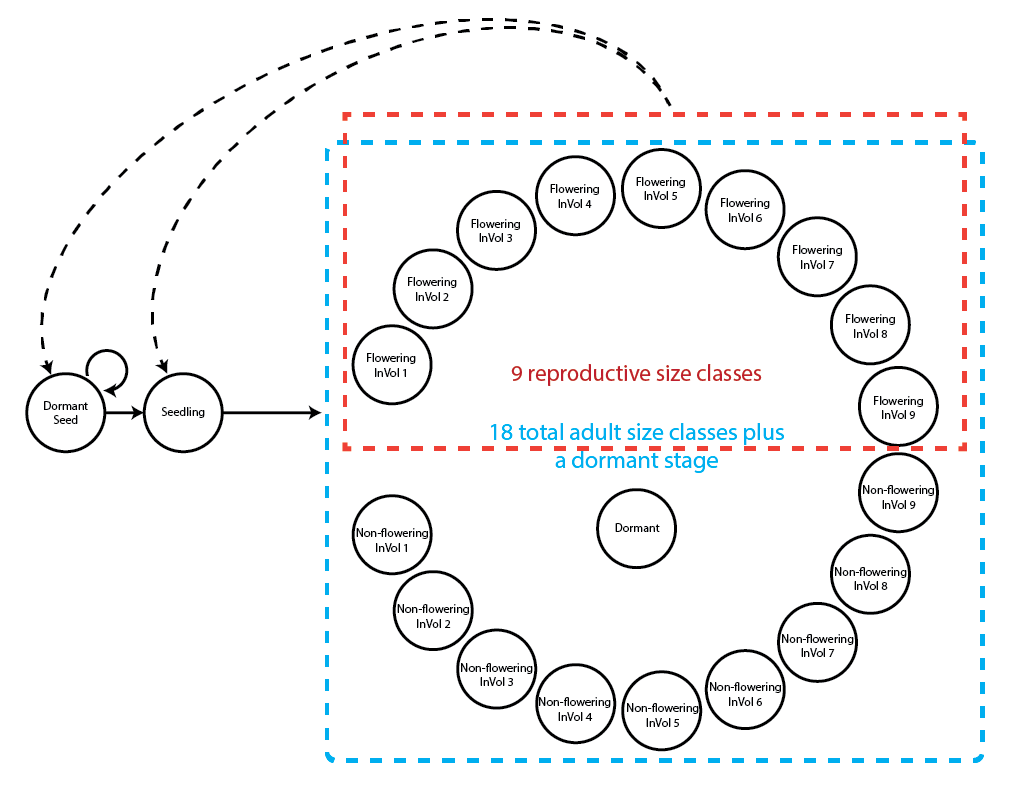Figure 8.1. Life history model of Lathyrus vernus using the log leaf volume as the size classification metric.

Our stageframe will include complete descriptions of all stages that occur in the dataset and in the life history model, with each stage defined uniquely. Since this object can be used for automated classification of individuals, all sizes, reproductive states, and other characteristics defining each stage in the dataset need to be accounted for explicitly. The final description of each stage occurring in the dataset must not completely overlap with any other stage also found in the dataset, although partial overlap is allowed and expected.

Before creating the stageframe, let’s explore the possible size variables. We will particularly look at summaries of the distribution of original and log sizes. Size here is given as leaf volume, and because this metric may have allometric relationships to vital rates, we will also look at log size.

summary(c(lathyrus$Volume88, lathyrus$Volume89, lathyrus$Volume90, lathyrus$Volume91))
#>    Min. 1st Qu.  Median    Mean 3rd Qu.    Max.    NA's
#>     1.8    14.7   123.0   484.2   732.5  7032.0    1248
summary(c(lathyrus$lnVol88, lathyrus$lnVol89, lathyrus$lnVol90, lathyrus$lnVol91))
#>    Min. 1st Qu.  Median    Mean 3rd Qu.    Max.    NA's
#>   0.600   2.700   4.800   4.777   6.600   8.900    1248

The upper summary shows the original size, while the lower line shows the size given in logarithmic terms. We should note the size minima and maxima, because we have been using 0 as the size of vegetatively dormant individuals. The lowest uncorrected size is 1.8, with a maximum of 7032. The minimum corrected size is 0.6, and the maximum corrected size is 8.9. Since the minimum corrected size is positive, and since the number of NAs has not increased (increased NAs would suggest that some unusable log sizes occur in the dataset), we are still able to use the log size value 0 as an indicator of vegetative dormancy. Note, however, that vegetative dormancy is currently included in the many NAs that occur in size variables in this dataset.

It can also help to take a look at plots of these distributions. We will plot raw and log volume.

par(mfrow=c(1,2))
plot(density(c(lathyrus$Volume88, lathyrus$Volume89, lathyrus$Volume90, lathyrus$Volume91), na.rm = TRUE), main = "", xlab = "Volume", bty = "n")
plot(density(c(lathyrus$lnVol88, lathyrus$lnVol89, lathyrus$lnVol90, lathyrus$lnVol91), na.rm = TRUE), main = "", xlab = "Log volume", bty = "n")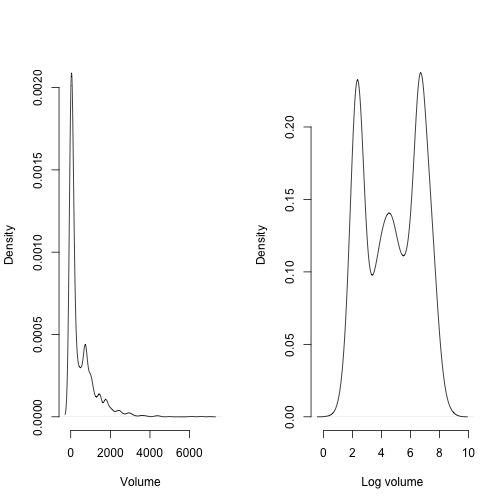Figure 8.2. Density plot of aboveground plant volume

The raw volume distribution is highly skewed. This might cause difficulty if we used raw size untransformed and with a Gaussian distribution. A Gamma distribution might be justified. However, we will use the log volume here, which looks ‘better’ than the raw volume distribution in the sense that it is closer to some semblance of a Gaussian distribution, mostly through an increased level of symmetry. We will assume that log volume is Gaussian-distributed and that the mean bears no relationship to the variance.

We will now develop a stageframe that incorporates the log volume of size. We will build this with vectors of the values describing each stage, always in the same order. Note that we are using the midpoint approach to determining the size bins here, using the default bin halfwidths of 0.5 (since all size bins are using this default, we do not include a binhalfwidth vector option in the sf_create() input). However, we could have used the sizemin and sizemax options to more deliberately set the size bin minima and maxima instead.

sizevector <- c(0, 4.6, 0, 1, 2, 3, 4, 5, 6, 7, 8, 9, 1, 2, 3, 4, 5, 6, 7, 8, 9)
stagevector <- c("Sd", "Sdl", "Dorm", "Sz1nr", "Sz2nr", "Sz3nr", "Sz4nr",
"Sz5nr","Sz6nr", "Sz7nr", "Sz8nr", "Sz9nr", "Sz1r", "Sz2r", "Sz3r", "Sz4r",
"Sz5r", "Sz6r", "Sz7r", "Sz8r", "Sz9r")
repvector <- c(0, 0, 0, 0, 0, 0, 0, 0, 0, 0, 0, 0, 1, 1, 1, 1, 1, 1, 1, 1, 1)
obsvector <- c(0, 1, 0, 1, 1, 1, 1, 1, 1, 1, 1, 1, 1, 1, 1, 1, 1, 1, 1, 1, 1)
matvector <- c(0, 0, 1, 1, 1, 1, 1, 1, 1, 1, 1, 1, 1, 1, 1, 1, 1, 1, 1, 1, 1)
immvector <- c(1, 1, 0, 0, 0, 0, 0, 0, 0, 0, 0, 0, 0, 0, 0, 0, 0, 0, 0, 0, 0)
propvector <- c(1, 0, 0, 0, 0, 0, 0, 0, 0, 0, 0, 0, 0, 0, 0, 0, 0, 0, 0, 0, 0)
indataset <- c(0, 1, 1, 1, 1, 1, 1, 1, 1, 1, 1, 1, 1, 1, 1, 1, 1, 1, 1, 1, 1)
binvec <- c(0, 4.6, 0.5, 0.5, 0.5, 0.5, 0.5, 0.5, 0.5, 0.5, 0.5, 0.5, 0.5, 0.5,
0.5, 0.5, 0.5, 0.5, 0.5, 0.5, 0.5)
minima <- c(0, 0, 1, 1, 1, 1, 1, 1, 1, 1, 1, 1, 1, 1, 1, 1, 1, 1, 1, 1, 1)
maxima <- c(NA, 1, NA, NA, NA, NA, NA, NA, NA, NA, NA, NA, NA, NA, NA, NA,
NA, NA, NA, NA, NA)
comments <- c("Dormant seed", "Seedling", "Dormant", "Size 1 Veg", "Size 2 Veg",
"Size 3 Veg", "Size 4 Veg", "Size 5 Veg", "Size 6 Veg", "Size 7 Veg",
"Size 8 Veg", "Size 9 Veg", "Size 1 Flo", "Size 2 Flo", "Size 3 Flo",
"Size 4 Flo", "Size 5 Flo", "Size 6 Flo", "Size 7 Flo", "Size 8 Flo",
"Size 9 Flo")
lathframeln <- sf_create(sizes = sizevector, stagenames = stagevector,
repstatus = repvector, obsstatus = obsvector, propstatus = propvector,
immstatus = immvector, matstatus = matvector, indataset = indataset,
binhalfwidth = binvec, minage = minima, maxage = maxima, comments = comments)
#lathframeln

#### Step 2a. Data standardization

Now we will standardize the dataset into historically-formatted vertical (hfv) format. Here, we will use the verticalize3() function, which creates historically-formatted vertical datasets from horizontally structured raw data, as below. We will also replace NAs in size and fecundity variables with zeros for modelsearch to work properly when we build models of vital rates, so we will now set NAas0 = TRUE. Some care needs to be taken with this last step, since some authors give missing values extra meaning not present in a value of zero. In our case, a missing value indicates that a plant was dead (both size and fecundity are missing), was alive but not sprouting (size was missing), or was alive but did not produce seed (fecundity was missing). In all cases, these NA values may be replaced by 0, because other variables indicate those conditions.

We also have two choices for use as our reproductive status and fecundity variables. The first choice, FCODE88 indicates whether a plant flowered. The second choice, Intactseed88, indicates the number of seed produced. Flowering plants typically have different demographic characteristics than non-flowering plants, either reflecting reproductive costs, or, conversely, because flowering plants might have more resources and hence higher survival than non-flowering plants. We should separate transitions among these two groups. So, let’s use FCODE88 to indicate reproductive status, and Intactseed88 to indicate fecundity.

Finally, note that in the input to the following function, we utilize a strictly repeating pattern of variable names arranged in the same order for each monitoring occasion. This arrangement allows us to enter only the first variable in each set, as long as noyears and blocksize are set properly and no gaps or shuffles appear in the dataset. The data management functions that we have created for lefko3 do not require such repeating patterns, but they do make the required input in the function much shorter and more succinct. To see a detailed summary of the resulting hfv dataset, remove full = FALSE from the summary_hfv() call. Note that prior to standardizing the dataset, we will create a new variable to code individual identity, since different plants in different subpopulations use the same identifiers.

lathyrus$indiv_id <- paste(lathyrus$SUBPLOT, lathyrus$GENET) lathvertln <- verticalize3(lathyrus, noyears = 4, firstyear = 1988, patchidcol = "SUBPLOT", individcol = "indiv_id", blocksize = 9, juvcol = "Seedling1988", sizeacol = "lnVol88", repstracol = "FCODE88", fecacol = "Intactseed88", deadacol = "Dead1988", nonobsacol = "Dormant1988", stageassign = lathframeln, stagesize = "sizea", censorcol = "Missing1988", censorkeep = NA, NAas0 = TRUE, censorRepeat = TRUE, censor = TRUE) summary_hfv(lathvertln, full = FALSE) #> #> This hfv dataset contains 2527 rows, 54 variables, 1 population, #> 6 patches, 1053 individuals, and 3 time steps. Before we move on to the next key steps in analysis, let’s take a closer look at fecundity. In this dataset, fecundity is mostly a count of intact seeds, and only differs in six cases where the seed output was estimated based on other models. To see this, try the following code. # Length of fecundity vaiable in t: length(lathvertln$feca2)
#>  2527
# Number of non-integer entries:
length(which(lathvertln$feca2 != round(lathvertln$feca2)))
#>  6

We see that we have quite a bit of fecundity data, and that it is overwhelmingly but not exclusively composed of integer values. The six non-integer values force us to make a decision - should we treat fecundity as a continuous variable, or round the values and treat it as a count variable? Here, we will round fecundity so that we can treat fecundity as a count variable in the analysis.

lathvertln$feca3 <- round(lathvertln$feca3)
lathvertln$feca2 <- round(lathvertln$feca2)
lathvertln$feca1 <- round(lathvertln$feca1)

Fecundity is now an integer variable, allowing us to use a count-based distribution. lefko3 currently allows six choices of count distributions: Poisson, negative binomial, zero-inflated Poisson, zero-inflated negative binomial, zero-truncated Poisson, and zero-truncated negative binomial. To assess which to use, we should first assess whether the mean and variance of the count are equal using a dispersion test. This test allows us to test whether the variance is greater than that expected under our mean value for fecundity using a chi-squared test. If it is not significantly different, then we may use some variant of the Poisson distribution. If the data are overdispersed, then we should use the negative binomial distribution. We should also test whether the number of zeroes is more than expected under these distributions, and make the distribution zero-inflated if so. Note that, because we have not excluded zeros from fecundity using reproductive status, we should not use a zero-truncated distribution.

We will use the hfv_qc() function to assess the quality of the data and decide on the correct distributions. We will use most of the input from the modelsearch() call that we will conduct later.

hfv_qc(data = lathvertln, vitalrates = c("surv", "obs", "size", "repst", "fec"),
juvestimate = "Sdl", indiv = "individ", year = "year2", age = "obsage")
#> Survival:
#>
#>   Data subset has 54 variables and 2246 transitions.
#>
#>   Variable alive3 has 0 missing values.
#>   Variable alive3 is a binomial variable.
#>
#>
#> Observation status:
#>
#>   Data subset has 54 variables and 2121 transitions.
#>
#>   Variable obsstatus3 has 0 missing values.
#>   Variable obsstatus3 is a binomial variable.
#>
#>
#> Primary size:
#>
#>   Data subset has 54 variables and 1916 transitions.
#>
#>   Variable sizea3 has 0 missing values.
#>   Variable sizea3 appears to be a floating point variable.
#>   1753 elements are not integers.
#>   The minimum value of sizea3 is 1.2 and the maximum is 8.8.
#>   The mean value of sizea3 is 5.099 and the variance is 3.093.
#>   The value of the Shapiro-Wilk test of normality is 0.9551 with P = 7.719e-24.
#>   Variable sizea3 differs significantly from a Gaussian distribution.
#>
#>   Variable sizea3 is fully positive, lacking even 0s.
#>
#>
#> Reproductive status:
#>
#>   Data subset has 54 variables and 1916 transitions.
#>
#>   Variable repstatus3 has 0 missing values.
#>   Variable repstatus3 is a binomial variable.
#>
#>
#> Fecundity:
#>
#>   Data subset has 54 variables and 599 transitions.
#>
#>   Variable feca2 has 0 missing values.
#>   Variable feca2 appears to be an integer variable.
#>
#>   Variable feca2 is fully non-negative.
#>
#>   Overdispersion test:
#>     Mean feca2 is 4.791
#>     The variance in feca2 is 70.14
#>     The probability of this dispersion level by chance assuming that
#>     the true mean feca2 = variance in feca2,
#>     and an alternative hypothesis of overdispersion, is 0
#>     Variable feca2 is significantly overdispersed.
#>
#>   Zero-inflation and truncation tests:
#>     Mean lambda in feca2 is 0.008302
#>     The actual number of 0s in feca2 is 334
#>     The expected number of 0s in feca2 under the null hypothesis is 4.973
#>     The probability of this deviation in 0s from expectation by chance is 0
#>     Variable feca2 is significantly zero-inflated.
#>
#>
#> Juvenile survival:
#>
#>   Data subset has 54 variables and 281 transitions.
#>
#>   Variable alive3 has 0 missing values.
#>   Variable alive3 is a binomial variable.
#>
#>
#> Juvenile observation status:
#>
#>   Data subset has 54 variables and 210 transitions.
#>
#>   Variable obsstatus3 has 0 missing values.
#>   Variable obsstatus3 is a binomial variable.
#>
#>
#> Juvenile primary size:
#>
#>   Data subset has 54 variables and 193 transitions.
#>
#>   Variable sizea3 has 0 missing values.
#>   Variable sizea3 appears to be a floating point variable.
#>   183 elements are not integers.
#>   The minimum value of sizea3 is 0.7 and the maximum is 4.1.
#>   The mean value of sizea3 is 2.307 and the variance is 0.2051.
#>   The value of the Shapiro-Wilk test of normality is 0.9273 with P = 3.278e-08.
#>   Variable sizea3 differs significantly from a Gaussian distribution.
#>
#>   Variable sizea3 is fully positive, lacking even 0s.
#>
#>
#> Juvenile reproductive status:
#>
#>   Data subset has 54 variables and 193 transitions.
#>
#>   Variable repstatus3 has 0 missing values.
#>   Variable repstatus3 is a binomial variable.
#>
#>
#> Juvenile maturity status:
#>
#>   Data subset has 54 variables and 210 transitions.
#>
#>   Variable matstatus3 has 0 missing values.
#>   Variable matstatus3 is a binomial variable.

All of the probability variables look like binomials, so we have no problem there. Size and juvenile size appear to be continuous variables that differ significantly from the assumptions of the Gaussian distribution. However, we will still use a Gaussian distribution in this case, for instructional purposes. Fecundity is a count variable with significant overdispersion and significantly more zeros than expected, so we should use a zero-inflated negative binomial distribution.

#### Step 2b. Provide supplemental information for matrix estimation

Matrix estimation functions in package lefko3 are made to work with supplement tables, which provide extra data for matrix estimation that is not included in the main demographic dataset. The supplemental() function provides a means of incorporating four additional kinds of data into MPM construction:

1. fixed transition values derived from other studies and added as constants to matrices,
2. proxy transition values when data for particular transitions do not exist and other, estimable transitions need to be used as proxies,
3. reproductive multipliers indicating which stages lead to the production of which stages, and at what level relative to estimated fecundity, as well as multipliers for proxy transitions, and
4. survival multipliers, in cases in which proxy survival transitions are used but must be set at elevated or reduced levels relative to the original transition.

Here, we will create an ahistorical supplemental table organizing some of these sorts of data. Each row refers to a specific transition. The first two of these transitions are set to specific probabilities, which are the probabilities of germination and seed dormancy, estimated from a separate study. The final two terms are fecundity multipliers, which mark which transitions correspond to fecundity and provide information on what multiple of fecundity estimated via linear modeling applies to each case. Note that type = 3 multipliers cannot be age-specific, while fecundity multipliers incorporated as type = 2 may be set to specific ages.

lathsupp2 <- supplemental(stage3 = c("Sd", "Sdl", "Sd", "Sdl"),
stage2 = c("Sd", "Sd", "rep", "rep"), givenrate = c(0.345, 0.054, NA, NA),
multiplier = c(NA, NA, 0.345, 0.054), type = c(1, 1, 3, 3),
stageframe = lathframeln, historical = FALSE, agebased = TRUE)
#lathsupp2

#### Step 3. Tests of history, and vital rate modeling

Next we will run the modelsearch function with the new vertical dataset. This function will develop our best-fit vital rate models for us. We provide descriptions of this function in the documentation, in some of the other vignettes, and in lefko3: a gentle introduction. Please see those materials for further information.

Here, we will create two ahistorical model sets. The first will be a model set for the entire population, without separating patches. The second will include patch as a random factor, and will thus allow us to explore patch dynamics as well as population dynamics. We will not create a historical set this time because we are producing an age x stage MPM only - lefko3 does not currently estimate or support historical age x stage MPMs. Note the use of age = "obsage" to identify the correct variable name, and test.age = TRUE to tell R to include age in the global models of vital rates.

lathmodelsln2 <- modelsearch(lathvertln, historical = FALSE,
approach = "mixed", suite = "main",
vitalrates = c("surv", "obs", "size", "repst", "fec"), juvestimate = "Sdl",
bestfit = "AICc&k", sizedist = "gaussian", fecdist = "negbin",
indiv = "individ", year = "year2", age = "obsage", test.age = TRUE,
year.as.random = TRUE, patch.as.random = TRUE, show.model.tables = TRUE,
fec.zero = TRUE, quiet = "partial")
#>
#> Developing global model of survival probability...
#>
#> Global model of survival probability developed. Proceeding with model dredge...
#>
#> Developing global model of observation probability...
#>
#> Global model of observation probability developed. Proceeding with model dredge...
#>
#> Developing global model of primary size...
#>
#> Global model of primary size developed. Proceeding with model dredge...
#>
#> Developing global model of reproduction probability...
#>
#> Global model of reproduction probability developed. Proceeding with model dredge...
#>
#> Developing global model of fecundity...
#>
#> Global model of fecundity developed. Proceeding with model dredge...
#>
#> Developing global model of juvenile survival probability...
#>
#> Global model of juvenile survival probability developed. Proceeding with model dredge...
#> Warning: Juvenile maturity status in time t+1 appears to be constant, and so will be set to constant.
#>
#> Developing global model of juvenile observation probability...
#>
#> Global model of juvenile observation probability developed. Proceeding with model dredge...
#>
#> Developing global model of juvenile primary size...
#>
#> Could not properly estimate a global model for juvenile size.
#> Warning: Juvenile reproductive status in time t+1 appears to be constant, and so will be set to constant.
#>
#> Finished selecting best-fit models.
lathmodelsln2p <- modelsearch(lathvertln, historical = FALSE,
approach = "mixed", suite = "main",
vitalrates = c("surv", "obs", "size", "repst", "fec"), juvestimate = "Sdl",
bestfit = "AICc&k", sizedist = "gaussian", fecdist = "negbin",
indiv = "individ", patch = "patchid", year = "year2", age = "obsage",
test.age = TRUE, year.as.random = TRUE, patch.as.random = TRUE,
show.model.tables = TRUE, fec.zero = TRUE, quiet = "partial")
#>
#> Developing global model of survival probability...
#>
#> Global model of survival probability developed. Proceeding with model dredge...
#>
#> Developing global model of observation probability...
#>
#> Global model of observation probability developed. Proceeding with model dredge...
#>
#> Developing global model of primary size...
#>
#> Global model of primary size developed. Proceeding with model dredge...
#>
#> Developing global model of reproduction probability...
#>
#> Global model of reproduction probability developed. Proceeding with model dredge...
#>
#> Developing global model of fecundity...
#>
#> Global model of fecundity developed. Proceeding with model dredge...
#>
#> Developing global model of juvenile survival probability...
#>
#> Global model of juvenile survival probability developed. Proceeding with model dredge...
#> Warning: Juvenile maturity status in time t+1 appears to be constant, and so will be set to constant.
#>
#> Developing global model of juvenile observation probability...
#>
#> Global model of juvenile observation probability developed. Proceeding with model dredge...
#>
#> Developing global model of juvenile primary size...
#>
#> Could not properly estimate a global model for juvenile size.
#> Warning: Juvenile reproductive status in time t+1 appears to be constant, and so will be set to constant.
#>
#> Finished selecting best-fit models.

#summary(lathmodelsln2)
#summary(lathmodelsln2p)

The output can be rather verbose, and so we have limited it with quiet = "partial". The function was developed to provide text marker posts of what the function is doing and what it has accomplished, as well as to show all warnings from all workhorse functions used. Because we used the mixed approach here, this includes warnings originating from estimating mixed linear models with package lme4 (Bates et al. 2015) and, in the case of fecundity, the package glmmTMB (Brooks et al. 2017). It also shows warnings originating from the dredge() function of package MuMIn (Bartoń 2014), which is the core function used in model building and AICc estimation. We encourage users to get familiar with interpreting these warnings and assessing the degree to which they impact their own analyses.

A look at the summaries shows that the best-fit models vary in complexity. Age is important in size, reproductive status, and fecundity in both the population-only model set as well as the patch model set. For example, the conditional model for fecundity is influenced by size and age in the current year, as well as by patch, individual identity, and year, while observation status is not influenced by age. We can see these models explicitly, as well as the model tables developed, by calling them directly from the lefkoMod object. The accuracy of many of our models is high, but some are mid-range and juvenile size is abysmally low, meaning that it is likely that we have not included some important factors accounting for variability in at least some of our vital rates.

#### Step 4. MPM estimation

Next, we will estimate the ahistorical sets of matrices. We will match the ahistorical age-by-stage matrix estimation function, aflefko2(), with the appropriate ahistorical input, including the ahistorical lefkoMod objects lathmodelsln2 and lathmodelsln2p. Model sets that include historical terms should not be used to create ahistorical matrices, since the coefficients in the best-fit models are estimated assuming a specific model structure that either relies on historical terms or does not. Historical vital rate models may yield biased results if used to construct ahistorical matrices. Also note that lefko3 does not currently allow the construction of historical age-by-stage MPMs. We will assume a prebreeding model, and set the maximum age to 3, per our dataset, but note that individuals may move past this age with continue = TRUE.

lathmat2age <- aflefko2(year = "all", stageframe = lathframeln,
modelsuite = lathmodelsln2, data = lathvertln, supplement = lathsupp2,
final_age = 3, continue = TRUE, reduce = FALSE)
lathmat2agep <- aflefko2(year = "all", patch = "all", stageframe = lathframeln,
modelsuite = lathmodelsln2p, data = lathvertln, supplement = lathsupp2,
final_age = 3, continue = TRUE, reduce = FALSE)

#summary(lathmat2age)
#summary(lathmat2agep)

The first model set led to the development of three matrices, reflecting the four years of data. The second model set led to the development of 18 matrices, reflecting four years and six patches. The quality control section gives us a sense of the amount of data used to model each vital rate, and also shows us that the survival-transition (U) matrices are composed entirely of proper probabilities yielding stage survival probability falling between 0.0 and 1.0. Matrix estimation protocols can sometimes create spurious values, such as stage survival greater than 1.0. Such values can occur for a variety of reasons, but the most common is the inclusion through a supplement table of externally-determined survival probabilities that are too high. Make sure to check your matrix column sums each time you estimate MPMs to prevent this problem. Survival greater than 1.0 can lead to strange effects on metrics of population dynamics.

We can get a sense of what these matrices look like by visualizing them. Let’s use the image3() function to look at the first image.

image3(lathmat2age, used = 1)
#> []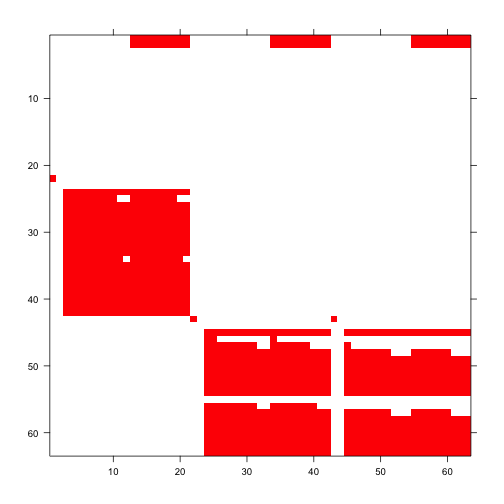Figure 8.3. Image of first A Matrix

The clear squares refer to zero elements, and the red elements refer to non-zero values corresponding to survival transitions and fecundity. The vast number of zeros may be surprising, but this matrix is a supermatrix organized by age first, with stage organizing within-age blocks. The first age is age 0, which cannot be adult, and age 1 corresponds to seedlings, leading to most non-zero elements in the adult portion. The adult block occurs from age 2, and this block can perpetuate indefinitely. The number of elements estimated is greater now than in the typical ahistorical MPM, because now we have added age as a major factor for analysis. This matrix is overwhelmingly composed of elements that must be 0, and so it is a rather sparse matrix ((1070.67 + 54) / 3969 = 28.3% of elements).

We can view the order of ages and stages using the agestages element of the lefkoMat object we produced, as below. Note that our matrix is 63 rows by 63 columns, and this object gives us the exact order used.

#lathmat2age$agestages Now let’s estimate the element-wise arithmetic mean matrices. The first lefkoMat object created will include a single mean matrix, while the second will include six patch-level mean matrices followed by a grand mean matrix, yielding seven matrices (remember to look at the labels element of the output to see the exact order of matrices). lathmat2mean <- lmean(lathmat2age) lathmat2pmean <- lmean(lathmat2agep) #summary(lathmat2mean) #summary(lathmat2pmean) #### Step 5. MPM analysis Now let’s estimate the deterministic population growth rate $$\lambda$$, and the stochastic population growth rate, $$a = \text{log} \lambda _{S}$$, in our age x stage MPM. We’ll plot them for comparison, making sure to take the natural exponent of the stochastic growth rate to make it comparable to $$\lambda$$. We will show the patch means and the grand population mean. lambda2 <- lambda3(lathmat2age) lambda2m <- lambda3(lathmat2mean) lambda2mp <- lambda3(lathmat2pmean) set.seed(42) sl2 <- slambda3(lathmat2age) #Stochastic growth rate sl2$expa <- exp(sl2$a) par(mfrow = c(1,2)) plot(lambda ~ year2, data = lambda2, ylim = c(0.90, 1.02),xlab = "Year", ylab = expression(lambda), type = "l", lty= 1, lwd = 2, bty = "n") abline(a = lambda2m$lambda, b = 0, lty = 2, lwd= 2, col = "orangered")
abline(a = sl2$expa, b = 0, lty = 2, lwd= 3, col = "darkred") legend("bottomleft", c("det annual", "det mean", "stochastic"), lty = c(1, 2, 2), col = c("black", "orangered", "darkred"), lwd = c(2, 2, 3), bty = "n") plot(lambda ~ patch, data = lambda2mp[1:6,], ylim = c(0.90, 1.02), xlab = "Patch", ylab = expression(lambda), type = "l", lty= 1, lwd = 2, bty = "n") abline(a = lambda2m$lambda, b = 0, lty = 2, lwd= 2, col = "orangered")
abline(a = sl2$expa, b = 0, lty = 2, lwd= 2, col = "darkred") legend("bottomleft", c("patch det mean", "pop det mean", "pop sto"), lty = c(1, 2, 2), col = c("black", "orangered", "darkred"), lwd = 2, bty = "n")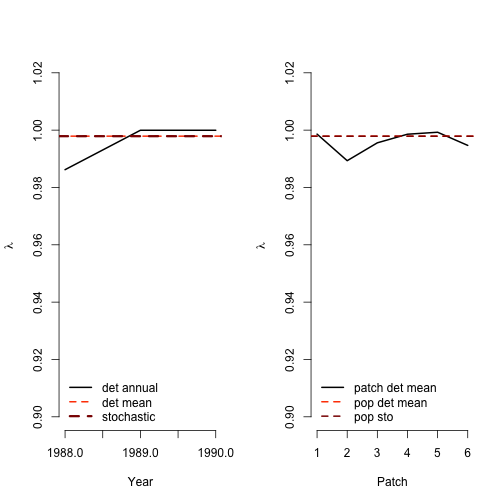Figure 8.4. Deterministic vs. stochastic lambda Deterministic and stochastic analyses suggest that the population and all patches are declining, with all years and patches associated with $$\lambda < 1$$ and $$a = \text{log} \lambda _{S} < 0$$. Qualitatively, they agree with the $$\lambda$$ and $$a = \text{log} \lambda _{S}$$ estimates from our other Lathyrus vignettes. Now let’s look at the stable stage distribution, using the population matrices rather than the patch matrices. The output for the ahistorical MPM is a data frame with matrix of origin, stage name, and stable stage proportion in each row. Because the default output for the stablestage3() function will give us the stable distribution of age-stages, we will collapse the output across age to produce a true stable stage distribution. ehrlen2ss <- stablestage3(lathmat2mean) ehrlen2ss_s <- stablestage3(lathmat2age, stochastic = TRUE, seed = 42) ss_props <- cbind.data.frame(ehrlen2ss$ss_prop, ehrlen2ss_s$ss_prop) names(ss_props) <- c("det", "sto") rownames(ss_props) <- paste(ehrlen2ss$age, ehrlen2ss$stage) det_dist <- apply(as.matrix(c(1:21)), 1, function(X) { ss_sum <- ss_props$det[X] + ss_props$det[21 + X] + ss_props$det[42 + X]
return(ss_sum)
})
sto_dist <- apply(as.matrix(c(1:21)), 1, function(X) {
ss_sum <- ss_props$sto[X] + ss_props$sto[21 + X] + ss_props$sto[42 + X] return(ss_sum) }) barplot(t(cbind.data.frame(det_dist, sto_dist)), beside = T, ylab = "Proportion", xaxt = "n", ylim = c(0, 0.2), col = c("black", "red"), bty = "n") text(cex=1, x=seq(from = 0.5, to = 3 * length(lathframeln$stage), by = 3),
y=-0.055, lathframeln$stage, xpd=TRUE, srt=45) legend("topright", c("deterministic", "stochastic"), col = c("black", "red"), pch = 15, bty = "n")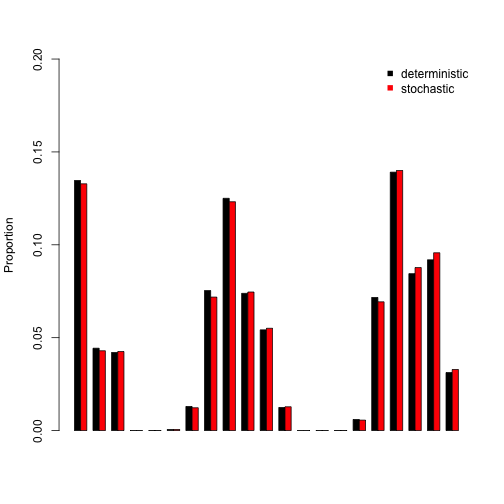Figure 8.5. Stable and long-run stage distribution The stable stage distribution shows high levels of dormant seeds and mid-sized adults. Small-sized adults comprise the smallest part of the stable age-stage structure. Deterministic and stochastic analyses show more or less the same results. Now let’s take look at the reproductive values. We’ll go straight to the plots, as with the stable stage distribution. ehrlen2rv <- repvalue3(lathmat2mean) ehrlen2rv_s <- repvalue3(lathmat2age, stochastic = TRUE, seed = 42) barplot(t(cbind.data.frame(ehrlen2rv$rep_value, ehrlen2rv_s$rep_value)), beside = T, ylab = "Relative rep value", xlab = "Age-Stage", ylim = c(0, 1.5), col = c("black", "red"), bty = "n") text(cex=0.5, y = -0.06, x = seq(from = 0, to = 2.98*length(rownames(ss_props)), by = 3), rownames(ss_props), xpd=TRUE, srt=45) legend("topleft", c("deterministic", "stochastic"), col = c("black", "red"), pch = 15, bty = "n")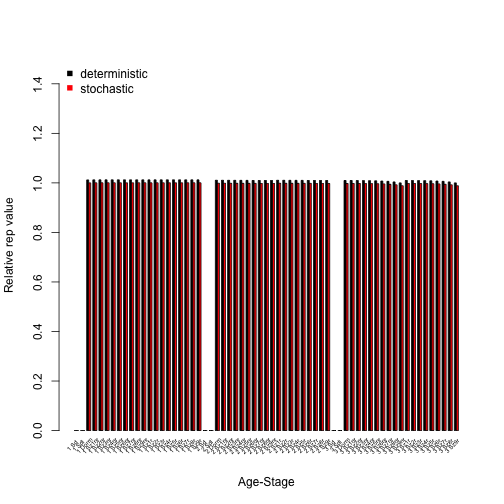Figure 8.6. Age-stage reproductive values We see reproductive values above 0 starting with dormant adults, and generally staying roughly equivalent with minor changes in ages 2 and 3. These patterns hold in both deterministic and stochastic analyses. We will next assess to which matrix elements $$\lambda$$ and $$\lambda_S$$ are most sensitive to changes in via deterministic and stochastic sensitivity analysis. lathmat2msens <- sensitivity3(lathmat2mean) set.seed(42) lathmat2msens_s <- sensitivity3(lathmat2age, stochastic = TRUE) # The highest deterministic sensitivity value: max(lathmat2msens$ah_sensmats[][which(lathmat2mean$A[] > 0)]) #>  0.1699623 # This value is associated with element: " which(lathmat2msens$ah_sensmats[] ==
max(lathmat2msens$ah_sensmats[][which(lathmat2mean$A[] > 0)]))
#>  3762

# The highest stochastic sensitivity value:
max(lathmat2msens_s$ah_sensmats[][which(lathmat2mean$A[] > 0)])
#>  0.4561818
# This value is associated with element:
which(as.matrix(lathmat2msens_s$ah_sensmats[]) == max(as.matrix(lathmat2msens_s$ah_sensmats[])[which(lathmat2mean$A[] > 0)])) #>  3888 The highest deterministic value appears to be associated with the transition from flowering size 6 adults in age 3 or higher to dormant adult (element 3762, in column 45, row 60). The stochastic sensitivity analysis suggests element 3888, which is associated with the transition from three year old flowering size 8 adults to dormant adult (column 62, row 45). Inspecting the sensitivity matrix (type lathmat2msens$ah_sensmats[] to view the full deterministic sensitivity matrix, or lathmat2msens_s$ah_sensmats[] to view the full stochastic sensitivity matrix) also shows that transitions near these elements are associated with rather high sensitivities. Let’s now assess the elasticity of $$\lambda$$ or $$\lambda_S$$ to matrix elements. lathmat2melas <- elasticity3(lathmat2mean) set.seed(42) lathmat2melas_s <- elasticity3(lathmat2age, stochastic = TRUE) # The highest deterministic elasticity value: max(lathmat2melas$ah_elasmats[][which(lathmat2mean$A[] > 0)]) #>  0.03229501 # The largest determnistic elasticity is associated with element: which(lathmat2melas$ah_elasmats[] == max(lathmat2melas$ah_elasmats[])) #>  3201 # The highest stochastic elasticity value: max(lathmat2melas_s$ah_elasmats[][which(lathmat2mean$A[] > 0)]) #>  0.2167045 # The largest stochastic elasticity is associated with element: which(as.matrix(lathmat2melas_s$ah_elasmats[]) == max(lathmat2melas_s$ah_elasmats[])) #>  3905 The deterministic analysis shows the highest elasticity associated with element 3201 (col 51, row 51, stasis as a size 6 non-reproductive 3yr old or older adult), while the stochastic analysis show that population growth rate is most elastic to element 3905, which is in column 62 and row 62 (transition from three year old or older size 8 reproductive adult to the same stage). Elasticity values can be treated as additive and sum to 1.0 within single matrices. This allows us to make interesting comparisons, such as to compare the elasticity of population growth rate to changes in transitions associated with specific stages. Let’s conduct such an analysis, and plot the results. elas_put_together <- cbind.data.frame(colSums(lathmat2melas$ah_elasmats[]),
Matrix::colSums(lathmat2melas_s$ah_elasmats[])) names(elas_put_together) <- c("det", "sto") rownames(elas_put_together) <- apply(as.matrix(c(1:dim(lathmat2melas$agestages))),
1, function(X) {
paste(lathmat2melas$agestages$stage[X], lathmat2melas$agestages$age[X])
})

barplot(t(elas_put_together), beside=T, names.arg = rep(NA,
length(rownames(elas_put_together))), ylab = "Elasticity", xlab = "Stage",
col = c("black", "orangered"), bty = "n")
text(cex=0.5, y = -0.007, x = seq(from = 0, to = 2.98*length(rownames(elas_put_together)),
by = 3), rownames(elas_put_together), xpd=TRUE, srt=45)
legend("topleft", c("deterministic", "stochastic"), col = c("black", "orangered"),
pch = 15, bty = "n")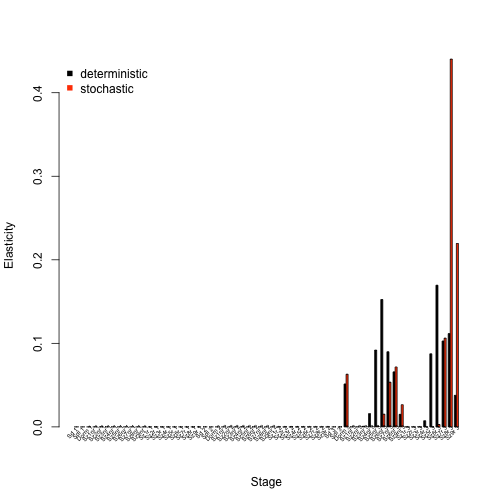Figure 8.7. Elasticities by age-stage

We see in the analysis above that in the deterministic case, the population growth rate is most elastic to changes in flowering and non-flowering mid-sized older adults. In the stochastic case, the population growth rate is most elastic to changes in the oldest and largest flowering adults. So, the differences are quite notable, and we would infer that environmental stochasticity makes a big impact here.

Our estimated elasticities can also be summarized by transition type. Let’s take a look at a plot, using the summary.lefkoElas() function to help us assess these transition types.

lathmat2m_sums <- summary(lathmat2melas)
lathmat2m_s_sums <- summary(lathmat2melas_s)

elas_sums_together <- cbind.data.frame(lathmat2m_sums$ahist[,2], lathmat2m_s_sums$ahist[,2])
names(elas_sums_together) <- c("det", "sto")
rownames(elas_sums_together) <- lathmat2m_sums$ahist$category

barplot(t(elas_sums_together), beside=T, ylab = "Elasticity", xlab = "Transition",
col = c("black", "orangered"), bty = "n")
legend("topright", c("deterministic", "stochastic"), col = c("black", "orangered"),
pch = 15, bty = "n")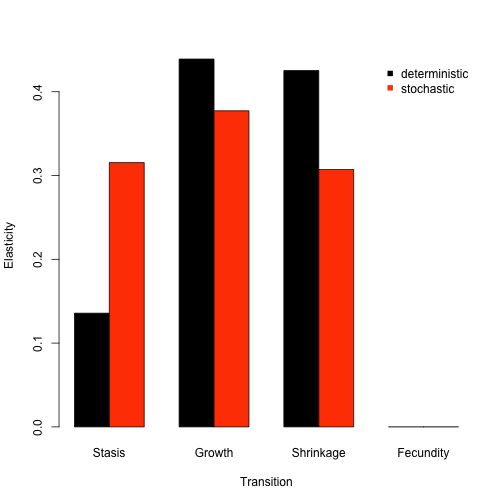Figure 8.8. Elasticities by transition type

We see in the plot above that deterministic and stochastic analyses agree strongly about the importance and influence of transition types to population growth rate. Specifically, the population growth rate is most strongly elastic in response to changes in growth transitions, and almost completely inelastic to changes in fecundity. Shrinkage is almost as influential as growth, and stasis is almost as important in stochastic analysis.

In addition to sensitivity and elasticity analyses, we can use package lefko3 to assess the actual impacts of demographic shifts or differences on the population growth rate. Two tools for this purpose are the life table response experiment (LTRE), and its stochastic versions the stochastic life table response experiment (sLTRE) and the small noise approximation LTRE (SNA-LTRE). All three are available via the ltre3() function. Below, we perform a standard deterministic LTRE to assess the impact of space on the population growth rate. This is done via a comparison of patch-level demography to the grand mean matrix, which is the default comparison if no reference matrices are provided. Remove the hashtag from the second line to see the full structure of the resulting object. Particularly, you will notice that the first element, ltre_det, is a list of six matrices. These matrices show the actual impact of the difference in elements between the respective patch-level matrix and the reference matrix (here, the grand population mean matrix) on the population growth rate $$\lambda$$. After this, we see similar structure to the input lefkoMat object, including the stageframe and order of matrices.

lathmat2m_ltre <- ltre3(lathmat2pmean)
#> Warning: Matrices input as mats will also be used in reference matrix calculation.
#> Using all refmats matrices in reference matrix calculation.
#lathmat2m_ltre

The sLTRE produces output that is a bit different. Here, we see two lists of matrices prior to the MPM metadata. The first, ltre_mean, is a list of matrices showing the impact of differences in mean elements between the patch-level temporal mean matrices and the reference temporal mean matrix. The second, ltre_sd, is a list of matrices showing the impact of differences in the temporal standard deviation of each element between the patch-level and reference matrix sets. In other words, while a standard LTRE shows the impact of changes in matrix elements on $$\lambda$$, the sLTRE shows the impacts of changes in the mean and the variability of matrix elements on $$\text{log} \lambda$$.

lathmat2m_sltre <- ltre3(lathmat2agep, stochastic = TRUE, rseed = 42)
#> Warning: Matrices input as mats will also be used in reference matrix calculation.
#> Using all refmats matrices in reference matrix calculation.
#lathmat2m_sltre

Let’s identify which age-stages exert the strongest impact on the population growth rate.

ltre_pos <- lathmat2m_ltre$cont_mean[] ltre_neg <- lathmat2m_ltre$cont_mean[]
ltre_pos[(ltre_pos < 0)] <- 0
ltre_neg[(ltre_neg > 0)] <- 0

sltre_meanpos <- lathmat2m_sltre$cont_mean[] sltre_meanneg <- lathmat2m_sltre$cont_mean[]
sltre_meanpos[(sltre_meanpos < 0)] <- 0
sltre_meanneg[(sltre_meanneg > 0)] <- 0

sltre_sdpos <- lathmat2m_sltre$cont_sd[] sltre_sdneg <- lathmat2m_sltre$cont_sd[]
sltre_sdpos[(sltre_sdpos < 0)] <- 0
sltre_sdneg[(sltre_sdneg > 0)] <- 0

ltresums_pos <- cbind(Matrix::colSums(ltre_pos), Matrix::colSums(sltre_meanpos),
Matrix::colSums(sltre_sdpos))
ltresums_neg <- cbind(Matrix::colSums(ltre_neg), Matrix::colSums(sltre_meanneg),
Matrix::colSums(sltre_sdneg))

ltre_as_names <- apply(as.matrix(c(1:length(lathmat2agep$agestages$stage))), 1,
function(X) {
paste(lathmat2agep$agestages$stage[X], lathmat2agep$agestages$age[X])
})
barplot(t(ltresums_pos), beside = T, col = c("black", "grey", "red"),
ylim = c(-0.010, 0.010))
barplot(t(ltresums_neg), beside = T, col = c("black", "grey", "red"), add = TRUE)
text(cex=0.5, y = -0.0095, x = seq(from = 0, to = 3.98*length(ltre_as_names),
by = 4), ltre_as_names, xpd=TRUE, srt=45)
legend("topleft", c("deterministic", "stochastic mean", "stochastic SD"),
col = c("black", "grey", "red"), pch = 15, bty = "n")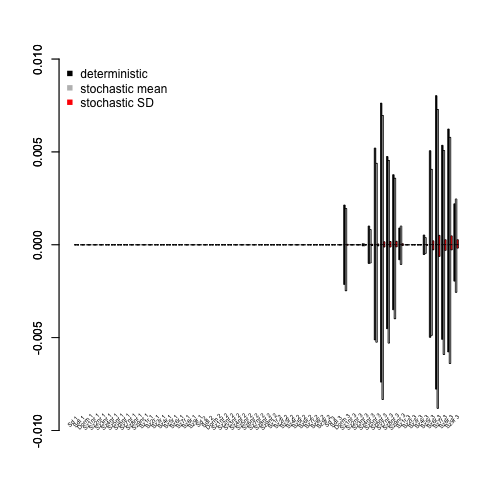Figure 8.9. LTRE contributions by age-stage

The output above shows that mid-sized non-flowering and flowering adults and seedlings exert strong influences on both $$\lambda$$ and $$\text{log} \lambda$$. This appears to be primarily through changes to the mean transition values, with only a small contribution due to changes in the variability of transition values in the stochastic case.

Finally, we will assess what transition types exert the greatest impact on population growth rate.

lathmat2m_ltre_summary <- summary(lathmat2m_ltre)
lathmat2m_sltre_summary <- summary(lathmat2m_sltre)

ltresums_tpos <- cbind(lathmat2m_ltre_summary$ahist_mean$matrix1_pos,
lathmat2m_sltre_summary$ahist_mean$matrix1_pos,
lathmat2m_sltre_summary$ahist_sd$matrix1_pos)
ltresums_tneg <- cbind(lathmat2m_ltre_summary$ahist_mean$matrix1_neg,
lathmat2m_sltre_summary$ahist_mean$matrix1_neg,
lathmat2m_sltre_summary$ahist_sd$matrix1_neg)

barplot(t(ltresums_tpos), beside = T, col = c("black", "grey", "red"),
ylim = c(-0.04, 0.04))
barplot(t(ltresums_tneg), beside = T, col = c("black", "grey", "red"),
abline(0, 0, lty = 3)
text(cex=0.85, y = -0.050, x = seq(from = 2,
to = 3.98*length(lathmat2m_ltre_summary$ahist_mean$category), by = 4),
lathmat2m_ltre_summary$ahist_mean$category, xpd=TRUE, srt=45)
legend("topleft", c("deterministic", "stochastic mean", "stochastic SD"),
col = c("black", "grey", "red"), pch = 15, bty = "n")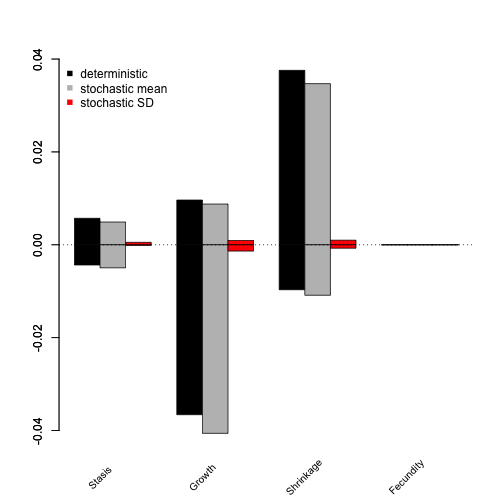Figure 8.10. LTRE contributions by transition type

The overall greatest impact on the population growth rate appears to come from growth and shrinkage transitions, with growth transitions having strongly negative influences in general.

LTREs and sLTREs are powerful tools, and more complex versions of both analyses exist. Particularly view our other online resources for the SNA-LTRE, and please consult Caswell (2001) and Davison et al. (2013) for more information. Users wishing to conduct these analyses should see our free e-manual called lefko3: a gentle introduction and other vignettes on the projects page of the Shefferson lab website.

## Acknowledgements

We are grateful to two anonymous reviewers whose scrutiny improved the quality of this vignette. The project resulting in this package and this tutorial was funded by Grant-In-Aid 19H03298 from the Japan Society for the Promotion of Science.

## Literature cited

Bartoń, Kamil A. 2014. MuMIn: Multi-Model Inference.” https://CRAN.R-project.org/package=MuMIn.
Bates, Douglas, Martin Maechler, Ben Bolker, and Steve Walker. 2015. “Fitting Linear Mixed-Effects Models Using Lme4.” Journal of Statistical Software 67 (1): 1–48. https://doi.org/10.18637/jss.v067.i01.
Brooks, Mollie E., Kasper Kristensen, Koen J. van Benthem, Arni Magnusson, Casper W. Berg, Anders Nielsen, Hans J. Skaug, Martin Machler, and Benjamin M. Bolker. 2017. glmmTMB Balances Speed and Flexibility Among Packages for Zero-Inflated Generalized Linear Mixed Modeling.” The R Journal 9 (2): 378–400. https://doi.org/10.32614/RJ-2017-066.
Caswell, Hal. 2001. Matrix Population Models: Construction, Analysis, and Interpretation. Second edition. Sunderland, Massachusetts, USA: Sinauer Associates, Inc.
Davison, Raziel, Florence Nicole, Hans Jacquemyn, and Shripad Tuljapurkar. 2013. “Contributions of Covariance: Decomposing the Components of Stochastic Population Growth in Cypripedium Calceolus.” The American Naturalist 181 (3): 410–20. https://doi.org/10.1086/669155.
Ehrlén, Johan. 2000. “The Dynamics of Plant Populations: Does the History of Individuals Matter?” Ecology 81 (6): 1675–84. https://doi.org/10.1890/0012-9658(2000)081[1675:TDOPPD]2.0.CO;2.